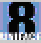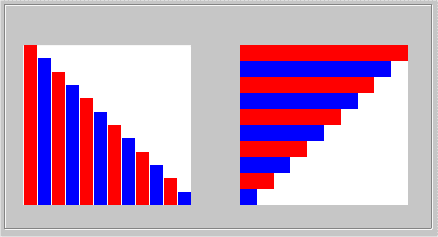## How to Create a Bar Graph in Uniface

 To create a bar graph in a Uniface form, you can use picture widgets containing glyphs with a uniform colour, which you scale horizontally and vertically depending on how tall and wide you want the bars to be. An example A form with a vertical and a horizontal bar graph will be created. We start with an empty form. For the vertical graph, an entity named GRAPH1 is painted with 12 occurrences repeated horizontally and for the horizontal graph an entity GRAPH2 is painted with 10 occurrences painted repeated vertically. The first occurrence in each entity is filled with a picture widget named VALUE. The colour of this widget is white - this will be the background colour of the graph. The data type of the field should be image(glyph). The picture widget in GRAPH1 must have Vertical alignment=bottom to start at the bottom of the graph and grow upwards. The checkbox "Preserve aspect" must be clicked off. In the horizontal graph, the picture widget must have Horizontal alignment=left if you want the bars to grow from the left to the right. The checkbox "Preserve aspect" must be clicked off.In the vertical graph, the horizontal scaling of all glyphs should be the same. The glyph is one "Uniface paint unit" wide but the field is two "paint units" wide so the horizontal scaling should be 200 (the value is entered in percent). The vertical scaling will vary to get different heights of the bars. The height of the glyph is one "Uniface paint unit" and the max height of the picture widget is 10 "paint units". This means that if the bar should fill the while height, its vertical scaling should be 1000 (10 * 100). The colour of the bars will alternate, so the red and the blue glyphs will be used alternating. In the horizontal graph, the vertical scaling of all glyphs should be the same. The glyph is one "Uniface paint unit" high and the field is also one "paint unit" high so the vertical scaling will be 100. In that case, you don't have to state the scaling of the glyph, but the code will do it for clarity. The horizontal scaling of the glyph will vary to give different lengths of the bars. The picture widgets will be filled with two glyphs, one uniformly red, RED_1 and the other uniformly blue, BLUE_1. The glyphs are of the same size, 1x1 Uniface "paint unit". Since this is not the same size in the vertical and horizontal direction, the glyphs are 7x16 pixels in size. The graphs are generated in a very simple way, with the vertical scale value getting smaller from the max value in the vertical graph and the horizontal scale value getting smaller in the horizontal graph.If you want labels on the graph axis, you must paint them as ordinary Uniface labels with text. The Code Needed to Create the Two Graphs In the execute-trigger of the form, two entries VERTICALGRAPH and HORIZONTALGRAPH are called to create the two graphs. The code is shown below. Since the horizontal scaling in the vertical graph is the same for all occurrences, \$properties of the field can be used to set the value once and for all. The scale value is set by setting the property HSCALE in the list \$properties. The vertical scaling varies for the occurrences and therefore \$fieldproperties must be used for this value. The scale value is set by setting the property VSCALE on the list \$fieldproperties. For the horizontal graph the opposite applies. ```entry VERTICALGRAPH variables numeric MAXVALUE float VSCALE string GLYPH endvariables MAXVALUE = 10 GLYPH = "RED_1" while (\$totocc(GRAPH1)<12) creocc "GRAPH1",-1 endwhile putitem/id \$properties("VALUE.GRAPH1"),"HSCALE", 200 ;Set horizontal scale setocc "GRAPH1",1 while (\$status > 0 & \$curocc(GRAPH1) <= 12) VALUE.GRAPH1=GLYPH if (GLYPH = "RED_1") ;Switch glyph for the next time GLYPH = "BLUE_1" else GLYPH = "RED_1" endif VSCALE = MAXVALUE * 100 * (13-\$curocc(GRAPH1))/12 putitem/id \$fieldproperties("VALUE.GRAPH1"),"VSCALE", VSCALE ;Set vertical scale setocc "GRAPH1", \$curocc(GRAPH1) + 1 endwhile end VERTICALGRAPH entry HORIZONTALGRAPH variables numeric MAXVALUE float HSCALE string GLYPH endvariables MAXVALUE = 24 GLYPH = "RED_1" while (\$totocc(GRAPH2) <10) creocc "GRAPH2",-1 endwhile putitem/id \$properties("VALUE.GRAPH1"),"VSCALE", 100 ;Not necessary setocc "GRAPH2",1 while (\$status > 0 & \$curocc(GRAPH2) <= 10) VALUE.GRAPH2=GLYPH if (GLYPH = "RED_1") GLYPH = "BLUE_1" else GLYPH = "RED_1" endif HSCALE = MAXVALUE * 100 * (11-\$curocc(GRAPH2))/10 putitem/id \$fieldproperties("VALUE.GRAPH2"),"HSCALE", HSCALE ;Set horizontal scale setocc "GRAPH2", \$curocc(GRAPH2) + 1 endwhile end HORIZONTALGRAPH```